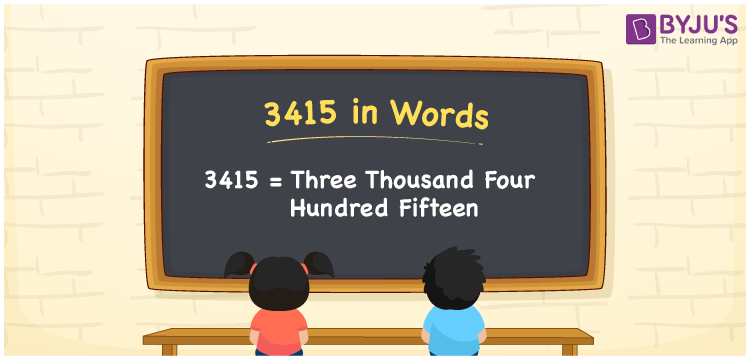# 3415 in Words

3415 in words is written as Three thousand four hundred fifteen. In both the International System of Numerals and the Indian System of Numerals, 3415 is written as Three thousand four hundred fifteen. The number 3415 is a Cardinal Number as it could represent some quantity. For example, “this video got 3415 likes”.

 3415 in Words Three thousand four hundred fifteen Three thousand four hundred fifteen in Number 3415

## 3415 in English Words

3415 in English words is read as “Three thousand four hundred fifteen”.## How to Write 3415 in Words?

To write 3415 in words, we shall use the place value chart. In the place value chart, put 3 in the thousands, 4 in the hundreds, 1 in the tens, and 5 in the ones, respectively. Let us make a place value chart to write the number 3415 in words.

 Thousands Hundreds Tens Ones 3 4 1 5

Thus, we can write the expanded form as

3 × Thousand + 4 × Hundred + 1 × Ten + 5 × One

= 3 × 1000 + 4 × 100 + 1 × 10 + 5 × 1

= 3000 + 400 + 10 + 5

= 3415

= Three thousand four hundred fifteen.

3415 is a natural number, the successor of 3414 and the predecessor of 3416.

3415 in words – Three thousand four hundred fifteen

• Is 3415 an odd number? – Yes
• Is 3415 an even number? – No
• Is 3415 a perfect square number? – No
• Is 3415 a perfect cube number? – No
• Is 3415 a prime number? – No
• Is 3415 a composite number? – Yes

## Frequently Asked Questions on 3415 in Words

Q1

### How to write 3415 in words?

3415 in words is written as Three thousand four hundred fifteen.
Q2

### How to write 3415 in the International and Indian System of Numerals?

In both, the system of numerals, 3415 in words, is written as Three thousand four hundred fifteen.
Q3

### How to write 3415 in a place value chart?

In the place value chart, write 3 in the thousands, 4 in the hundreds, 1 in the tens, and 5 in the ones, respectively.